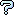All about flooble | fun stuff | Get a free chatterbox | Free JavaScript | Avatarsperplexus dot infoRadically confusing (Posted on 2005-05-14)Simplify the product A*B*C*D*E*F

A = (√2)
B = (√(2-√2))
C = (√(2-√(2+√2)))
D = (√(2-√(2+√(2+√2))))
E = (√(2-√(2+√(2+√(2+√2)))))
F = (√(2+√(2+√(2+√(2+√2)))))

 See The Solution Submitted by Jer Rating: 1.4286 (7 votes)Comments: ( Back to comment list | You must be logged in to post comments.)re(2): it is two easy| Comment 8 of 15 |(In reply to re: it is two easy by Charlie)

Are the minus signs in B, C and D supposed to be plus signs? I think then it would work out as Ady TZIDON initially said.

... in which case, the formulae for E and F should probably be reversed, so as to put the odd one (negative sign) at the bottom.

Edited on May 16, 2005, 3:40 am
 Posted by Charlie on 2005-05-16 03:39:04Please log in:

 Search: Search body:
Forums (0)